Book Author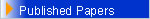• Vector Calculus and Analytic Geometry , 1980 , Evos Publ. , Greece
• Mixed type Equations and Maximum Principles in fluid dynamics , 1983 , Greece
• Probability Theory and Statistics , 1989 , Symmetry Publ. , Greece
• Number Theory , 1991 , Symmetry Publ. , Greece
• Linear Algebra and Linear Programming , 1993 , Symmetry Publ., Greece
• Mathematical Analysis : Differential Calculus , Vol. I , 1994, Symmetry Publ. , Greece
• Mathematical Analysis : Integral Calculus , Vol. II , 1995 , Symmetry Publ. , Greece
• Mathematical Analysis : Multivariable Calculus , Vol. III , 1996, Symmetry Publ. , Greece
• Applied Analysis : Differential Geometry , Vol. I , 2002, Symmetry Publ. , Greece
• Applied Analysis : Partial Differential Equations , Vol. II , 2003, Symmetry Publ. , Greece
• Lecture Notes on Mixed Type Partial Differential Equations, World Scientific , 144 pp,1990
• Counter Examples in Differential Equations and Related Topics, World Scientific , 192 pp,1991

Selected Citations :

o       W. F. Ames ( G I T ) – for the Mathematical Reviews , AMS :

Any analyst is always concerned about good counterexamples to use in lectures and research. Here is a book with a collection of counterexamples in the areas of continuity, differentiability, extrema, existence, uniqueness, stability, regularity, periodicity, etc. They have been collected from a variety of books and journals. The collection should prove useful to analysts interested in the main topic of differential equations. There is a reasonable bibliography and both a subject and an author index. This is a valuable book for students and researchers alike.

o       G.C.Rota (M.I.T.) for the World Scientific :

The author knows his business : he has chosen the right counterexamples at the right time. The section on plane autonomous systems is particularly felicitous, and should be consulted by whoever teaches elementary differential equations ( some of the examples are really cute and we will use them in our 400-student course next year ). Why can't mathematicians write more books like this one ?

BOOKS [In Greek]
PUBLICATIONS SYMMETRY, ATHENS
Author: John M. Rassias

ΒΙΒΛΙΑ [ΣΤΑ ΕΛΛΗΝΙΚΑ]
ΕΚΔΟΣΕΙΣ ΣΥΜΜΕΤΡΙΑ, ΑΘΗΝΑ
Συγγραφέας: Ιωάννης Μ. Ρασσιάς

ΜΑΘΗΜΑΤΙΚΗ ΑΝΑΛΥΣΗ Τόμος Ι - ΔΙΑΦΟΡΙΚΟΣ ΛΟΓΙΣΜΟΣ
(MATHEMATICAL ANALYSIS Volume I-DIFFERENTIAL CALCULUS)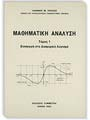ΡΑΣΣΙΑΣ ΙΩΑΝΝΗΣ Μ. (RASSIAS  JOHN Μ.) Σελ. /pp. 1-212

ΜΑΘΗΜΑΤΙΚΗ ΑΝΑΛΥΣΗ Τόμος ΙI - ΟΛΟΚΛΗΡΩΤΙΚΟΣ  ΛΟΓΙΣΜΟΣ
(MATHEMATICAL ANALYSIS  Volume II – INTEGRAL CALCULUSΡΑΣΣΙΑΣ ΙΩΑΝΝΗΣ Μ. (RASSIAS  JOHN Μ.) Σελ. /pp. 213-470

ΜΑΘΗΜΑΤΙΚΗ ΑΝΑΛΥΣΗ Τόμος ΙΙΙ  - ΛΟΓΙΣΜΟΣ ΠΟΛΛΩΝ ΜΕΤΑΒΛΗΤΩΝ
(MATHEMATICAL ANALYSIS  Volume  III –CALCULUS OF SEVERAL VARIABLES)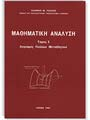ΡΑΣΣΙΑΣ ΙΩΑΝΝΗΣ Μ. (RASSIAS  JOHN Μ.) Σελ. /pp. 471-786

ΕΦΑΡΜΟΣΜΕΝΗ ΑΝΑΛΥΣΗ Τόμος Ι - ΔΙΑΦΟΡΙΚH  ΓΕΩΜΕΤΡΙΑ
(APPLIED ANALYSIS Volume  I –DIFFERENTIAL GEOMETRY)ΡΑΣΣΙΑΣ ΙΩΑΝΝΗΣ Μ. (RASSIAS  JOHN Μ.) Σελ. /pp. 1-288

ΕΦΑΡΜΟΣΜΕΝΗ ΑΝΑΛΥΣΗ Τόμος ΙΙ  - ΜΕΡΙΚΕΣ  ΔΙΑΦΟΡΙΚΕΣ ΕΞΙΣΩΣΕΙΣ
(APPLIED ANALYSIS  Volume  II – PARTIAL DIFFERENTIAL EQUATIONS)ΡΑΣΣΙΑΣ ΙΩΑΝΝΗΣ Μ. (RASSIAS  JOHN Μ.) Σελ. /pp. 289-701

ΓΡΑΜΜΙΚΗ   ΑΛΓΕΒΡΑ ΚΑΙ ΓΡΑΜΜΙΚΟΣ ΠΡΟΓΡΑΜΜΑΤΙΣΜΟΣ
(LINEAR ALGEBRA  AND  LINEAR PROGRAMMINGΡΑΣΣΙΑΣ ΙΩΑΝΝΗΣ Μ. (RASSIAS  JOHN Μ.) Σελ. /pp. 1-269

ΘΕΩΡΙΑ ΑΡΙΘΜΩΝ
(NUMBER THEORY)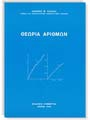ΡΑΣΣΙΑΣ ΙΩΑΝΝΗΣ Μ. (RASSIAS JOHN Μ.) Σελ. /pp. 1-377

ΘΕΩΡΙΑ ΠΙΘΑΝΟΤΗΤΩΝ ΚΑΙ ΣΤΑΤΙΣΤΙΚΗΣ
(THEORY OF PROBABILITY AND STATISTICS)ΡΑΣΣΙΑΣ ΙΩΑΝΝΗΣ Μ. (RASSIAS JOHN Μ.) Σελ. /pp. 1-489

BOOKS [In English]
Author : John Michael Rassias

MIXED TYPE PARTIAL DIFFERENTIAL EQUATIONS

Wold Scientific Publishing Co. Pte. Ltd, Singapore
1990, 1-144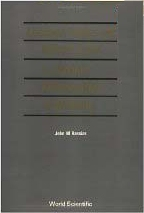Contents:
• The Dynamical Equation of Mixed Type
• The Tricomi Problem
• Regularity of Solutions (in the sense of Tricomi)
• Fundamental Idea of Tricomi
• The Gellerstedt Problem
• The Frankl Problem
• Quasi-Regularity of Solutions (in the sense of Protter)
• The a, b, c Energy Integral Method
• Weak (or Strong) Solutions in the Classical Sense
• Well Posedness
• A Selection of New Results
• Open Problems
• Basic Books

COUNTER EXAMPLES IN DIFFERENTIAL EQUATIONS
AND RELATED TOPICS

Wold Scientific Publishing Co. Pte. Ltd, Singapore
1991, 1-192Contents:
• Continuity and Linearity
• Differentiability and Analyticity
• Extrema
• Existence
• Uniqueness
• Stability
• Singularities
• Dynamical Systems
• Integral Equations
• Other Topics
• Open Problems